Published: 30 September 2016

# An improved Fourier series method for vibration analysis of moderately thick annular and circular sector plates subjected to elastic boundary conditions

Dongyan Shi2
Zarnab Hina3
Syed M. Aftab4
Hafiz M. Waqas5
1, 2, 4, 5College of Mechanical and Electrical Engineering, Harbin Engineering University, Harbin, China
3College of Information and Communication Engineering, Harbin Engineering University, Harbin, China
Corresponding Author:
Views 265

#### Abstract

In this paper, an improved Fourier series method is presented for vibration analysis of moderately thick annular and circular sector plates subjected to general elastic boundary conditions along its edges. In literature, annular and circular sector plates subjected to classical boundary conditions have been studied in detail however in practical engineering applications the boundary conditions are not always classical in nature. Therefore, study of vibration response of these plates subjected to general elastic boundary conditions is far needed. In the method presented, artificial boundary spring technique has been employed to simulate the general elastic boundary conditions and first order shear deformation theory has been employed to formulate the theoretical model. Irrespective of the boundary conditions, each of the displacement function is expressed as a new form of trigonometric expansion with accelerated convergence. Rayleigh-Ritz method has been employed to determine the expansion coefficients. Unlike most of the studies on vibration analysis of moderately thick annular sector plates, the present method can be universally applied to a wide range of vibration problems involving different boundary conditions, varying material and geometric properties without modifying the solution algorithms and procedure. The effectiveness, reliability and accuracy of the present method is fully demonstrated and verified by several numerical examples. Bench mark solutions for moderately thick annular sector and circular plates under general elastic boundary conditions are also presented for future computational methods.

## 1. Introduction

Annular, circular and their sector parts are key structural components that are used in various engineering fields like civil, marine, aerospace and mechanical engineering. Due to different geometrical shapes of these structures, they have been analyzed separately using different solution techniques. Most of the initial research on these plates was done using Classical plate theory (CPT) in which the shear deformation and rotary inertia was neglected which in turn limited its application on moderately thick and thick plates. Later a lot of theories were proposed incorporating the shear deformation and rotary inertia which resulted in an increase in accuracy of the results for moderately thick and thick annular and circular plates. These theories’ have been well explained in Leissa’s book on vibration of plates.

Different methods have been employed by various researchers to study the vibration characteristics of annular and circular plates subjected to different boundary conditions. However, a few prominent studies related to these plates are highlighted here in this manuscript. Employing the Mindlin plate theory on thick sector plates, Guruswamy et al.  studied the dynamic response of these plates by proposing a sector finite element. Fully clamped boundary conditions were employed on all edges. Taking the effect of shear deformation in thickness direction, another study was performed by Soni et al.  on axisymmetric non uniform circular disks. In their research they employed Chebyshev collocation technique to study the vibration characteristics of these plates. In another study Rayleigh-Ritz method was employed by Liew et al. on circular plates with multiple internal ring supports. Later in another study they studied the characteristics of these plates subjected to in-plane pressure [3-4].

Using three dimensional finite strip model, thick and thin sector plates subjected to various combinations of classical boundary condition were analyzed by Cheung et al. . The integral equation technique and finite strip method was employed by Sirinivasan et al. and Misuzawa et al. respectively to study the vibration characteristics of Mindlin annular sector plates [6-7]. Employing the Mindlin plate theory and differential quadrature method, Liu et al. studied the effect of sector angle and thickness to radius ratio on the vibration characteristics of moderately thick sector plates . Later they extended the same differential quadrature method to annular sector plates having shear deformation subjected to different combinations of classical boundary conditions . In another study, the same differential quadrature method was employed on solid circular plates with variable thickness in radial direction and subjected to elastic boundary conditions by Wu et al. . Similarly, Xiang et al. employed domain decomposition technique and studied the vibration response of circular plates with stepped thickness variation .

Using Rayleigh-Ritz method So et al.  studied the vibration characteristics of annular and circular plates by employing three dimensional elasticity theory. A similar three dimensional study was performed by Hashemi et al. on annular sector plates resting on elastic foundations. He employed polynomial-Ritz approach and studied the vibration characteristics for different sets of classical boundary conditions . In another study similar polynomial-Ritz model was presented by Liew et al. to investigate the effect of boundary conditions and thickness on the vibration characteristics of annular plates . In another prominent three dimensional study Chebyshev-Ritz technique was employed on circular and annular plates by Zhou et al. to study the vibration characteristics of these plates . In plane vibrations of circular plates subjected to different boundary conditions were investigated by various researchers using different solution techniques [16-17]

From the studies mentioned above it can be seen that most of the previous studies on annular and circular plates were limited to classical boundary conditions which includes free, simply supported, clamped or combination of these. However, in practical engineering applications the boundary conditions are not always classical in nature. Therefore, the development of an analytical method universally dealing with arbitrary boundary conditions was much needed. An improved Fourier series method was developed for vibration analysis of beams and plates by Li [18-22]. Later Xianjie Shi et al. extended this method to thin annular plates to study its vibration characteristics [23-26]. The main objective of this study is to realize and extend the same generalized Fourier series method to study the vibration analysis of Mindlin annular sector and circular sector plates under various boundary conditions including the general elastic restraints.

## 2. Theoretical formulation

### 2.1. Model description

Consider an annular sector plate of constant thickness $h$, inner radius $a$, outer radius $b$ and width $R$ in radial direction as shown in Fig. 1. The plate geometry and dimensions are defined in the cylindrical coordinate system $\left(r,\varphi ,z\right)$. A local coordinate system $\left(s,\varphi ,z\right)$ is also shown in Fig. 1. The radial and thickness coordinates $s$ and $z$ are measured normally from the inner edge and mid plane of the annular sector plate respectively whereas $\varphi$ is the circumferential angle. Four sets of distributed springs (one translational and two rotational) of arbitrary stiffness values are attached at each edge to simulate arbitrary boundary conditions. All the classical sets of boundary conditions can easily be achieved by varying the stiffness value of each spring from zero to an infinitely large number i.e. 1014.

The domain of the annular sector plate can be defined as:

1
$0\le s\le R,\mathrm{}\mathrm{}\left(R=b-a\right),\mathrm{}\mathrm{}\mathrm{}\mathrm{}\mathrm{}-\frac{h}{2}\le z\le \frac{h}{2},\mathrm{}\mathrm{}\mathrm{}\mathrm{}\mathrm{}0\le \varphi <2\pi ,$

The relationship between local and global coordinate system can be expressed as:

2
$s=r-a.$

The material of the plate is assumed to be isotropic with material density $\rho$, young’s modulus $E$ and Poisson ratio $\nu$. It should be noted that a circular sector plate can be defined as a special case of an annular sector plate if the inner radius ‘$a$’ is set either equal to 0 or to a very small number say 0.00001.

The displacement field of Mindlin annular sector plate in cylindrical coordinates is given by:

3
${u}_{r}\left(r,\varphi ,z,t\right)={u}_{r}\left(r,\varphi ,z\right)+z{\theta }_{r}\left(r,\varphi ,t\right),$
${u}_{\varphi }\left(r,\varphi ,z,t\right)={u}_{\varphi }\left(r,\varphi ,z\right)+z{\theta }_{\varphi }\left(r,\varphi ,t\right),$
$w\left(r,\varphi ,z,t\right)={w}_{o}\left(r,\varphi ,t\right),$

where $z$ is the thickness coordinate, ${u}_{r}$ and ${u}_{\varphi }$ are displacements of the mid plane in $r$ and $\varphi$ directions, respectively, $w$ is the transverse displacement. ${\theta }_{r}$ and ${\theta }_{\varphi }$ are the rotation functions of the middle surface and $t$ is the time. Assuming the plain stress distribution in accordance with Hooks law, the stress resultants are obtained for Mindlin annular plate by integrating the stresses as shown below:

4
${M}_{rr}=\underset{-h/2}{\overset{h/2}{\int }}{\sigma }_{rr}zdz=\underset{-h/2}{\overset{h/2}{\int }}\frac{E}{\left(1-{\nu }^{2}\right)}\left({\epsilon }_{rr}+\nu {\epsilon }_{\varphi \varphi }\right)zdz=D\left[\frac{\partial {\theta }_{r}}{\partial r}+\frac{\nu }{r}\left({\theta }_{r}+\frac{\partial {\theta }_{\varphi }}{\partial \varphi }\right)\right],$
${M}_{\varphi \varphi }=\underset{-h/2}{\overset{h/2}{\int }}{\sigma }_{\varphi \varphi }zdz=\underset{-h/2}{\overset{h/2}{\int }}\frac{E}{1-{\nu }^{2}}\left({\epsilon }_{\varphi \varphi }+\nu {\epsilon }_{rr}\right)zdz=D\left[\frac{1}{r}\left({\theta }_{r}+\frac{\partial {\theta }_{\varphi }}{\partial \varphi }\right)+\nu \left(\frac{\partial {\theta }_{r}}{\partial r}\right)\right],$
${M}_{r\varphi }=\underset{-h/2}{\overset{h/2}{\int }}{\tau }_{r\varphi }zdz=\underset{-h/2}{\overset{h/2}{\int }}G{\gamma }_{r\varphi }zdz=D\left(\frac{1-\nu }{2}\right)\left[\frac{1}{r}\left(\frac{\partial {\theta }_{r}}{\partial \varphi }-{\theta }_{\varphi }\right)+\frac{\partial {\theta }_{\varphi }}{\partial r}\right],$
${Q}_{rr}={K}^{2}\underset{-h/2}{\overset{h/2}{\int }}{\tau }_{rz}dz={K}^{2}Gh\left[{\theta }_{r}+\frac{\partial {w}_{o}}{\partial r}\right],$
${Q}_{\varphi \varphi }={K}^{2}\underset{-h/2}{\overset{h/2}{\int }}{\tau }_{\varphi z}dz={K}^{2}Gh\left[{\theta }_{\varphi }+\frac{1}{r}\frac{\partial {w}_{o}}{\partial \varphi }\right],$

where ${M}_{rr}$, ${M}_{\varphi \varphi }$ and ${M}_{r\varphi }$ are the bending moments per unit length of the plate, ${Q}_{rr}$ and ${Q}_{\varphi \varphi }$ are the transverse shear forces per unit length of the plate, ${\sigma }_{rr}$, ${\sigma }_{\varphi \varphi }$ are the normal stresses, and ${\tau }_{r\varphi }$, ${\tau }_{rz}$ and ${\tau }_{rz}$ are the shear stresses, $h$ is the plate thickness, $E$ is the modulus of elasticity, $G$ is the shear modulus, $\nu$ is the Poisson ratio, $D=E{h}^{3}/12\left(1-{\nu }^{2}\right)$ is the flexural rigidity and ${K}^{}={\pi }^{2}/12$ is the shear correction factor to compensate for the error in assuming the constant shear stress throughout the plate thickness.

The equation of motion of Mindlin plates in $\left(r,\varphi ,z\right)$ is given by:

5
$\frac{\partial {M}_{rr}}{\partial r}+\frac{1}{r}\frac{\partial {M}_{r\varphi }}{\partial \varphi }+\frac{1}{r}\left({M}_{rr}-{M}_{\varphi \varphi }\right)-{Q}_{rr}=\frac{\rho {h}^{3}}{12}\left(\frac{{\partial }^{2}{\theta }_{r}}{\partial {t}^{2}}\right),$
$\frac{\partial {M}_{r\varphi }}{\partial r}+\frac{1}{r}\frac{\partial {M}_{\varphi }}{\partial \varphi }+\frac{2}{r}{M}_{r\varphi }-{Q}_{\varphi \varphi }=\frac{\rho {h}^{3}}{12}\left(\frac{{\partial }^{2}{\theta }_{\varphi }}{\partial {t}^{2}}\right),$
$\frac{\partial {Q}_{rr}}{\partial r}+\frac{1}{r}\frac{\partial {Q}_{\varphi \varphi }}{\partial \varphi }+\frac{{Q}_{rr}}{r}=\rho h\frac{{\partial }^{2}{w}_{o}}{\partial {t}^{2}}.$

Fig. 1Mindlin sector plate geometry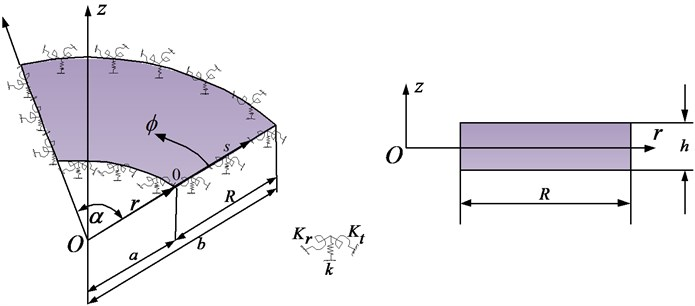### 2.2.1. Selection of admissible displacement function

Assume that the displacement field of Mindlin annular sector plate in local coordinate system $\left(s,\varphi ,z\right)$ is defined by the following series:

6
${\theta }_{s\left(s,\varphi \right)}=\sum _{m=n=-2}^{\infty }{A}_{mn}{\phi }_{m}\left(s\right){\phi }_{n}\left(\varphi \right),\left(r=s+a\right),$
${\theta }_{\varphi \left(s,\varphi \right)}=\sum _{m=n=-2}^{\mathrm{\infty }}{B}_{mn}{\phi }_{m}\left(s\right){\phi }_{n}\left(\varphi \right),$
${w}_{o\left(s,\varphi \right)}=\sum _{m=n=-2}^{\mathrm{\infty }}{C}_{mn}{\phi }_{m}\left(s\right){\phi }_{n}\left(\varphi \right),$

where:

${\phi }_{m}\left(s\right)=\left\{\begin{array}{l}\mathrm{c}\mathrm{o}\mathrm{s}{\lambda }_{m}\left(s\right),\mathrm{}\mathrm{}\mathrm{}m\ge 0\\ \mathrm{s}\mathrm{i}\mathrm{n}{\lambda }_{m}\left(s\right),\mathrm{}\mathrm{}\mathrm{}\mathrm{}m<0\end{array}\right\,{\phi }_{n}\left(\varphi \right)=\left\{\begin{array}{l}\mathrm{c}\mathrm{o}\mathrm{s}{\lambda }_{n}\left(\varphi \right),\mathrm{}\mathrm{}\mathrm{}n\ge 0\\ \mathrm{s}\mathrm{i}\mathrm{n}{\lambda }_{n}\left(\varphi \right),\mathrm{}\mathrm{}\mathrm{}\mathrm{}n<0\end{array}\right\,$

and ${\lambda }_{m}=m\pi /R\text{,}$${\lambda }_{n}=n\pi /\alpha$ and ${A}_{mn}\text{,}$${B}_{mn}\text{,}$${C}_{mn}$ denotes the Fourier series expansion coefficients. The sine terms in the equations Eq. (6) are introduced to overcome the potential discontinuities (convergence problem) of the displacement function, along the edges of the plate, when it is periodically extended and sought in the form of trigonometric series expansion. The addition of these auxiliary functions in the admissible functions plays an important role in the convergence and accuracy of the present method or in other words the elimination of potential discontinuities at the ends or elimination of Gibbs effect.

In order to illustrate this, take a beam problem for example. The governing equations for free vibration of a general supported Euler beam is obtained as:

7
$D\frac{{\partial }^{4}w\left(x\right)}{\partial {x}^{4}}-\rho A{\omega }^{2}w\left(x\right)=0,$

where $D$, $\rho$ and $A$ are, respectively, the flexural rigidity, the mass density and the cross sectional area of the beam, and $\omega$ is frequency in radian. From Eq. (7) it can be observed that the displacement solution $w\left(x\right)$ on a beam of length $L$ is required to have up to the fourth derivatives, that is, $w\left(x\right)\in {C}^{3}$. In general, the displacement function $w\left(x\right)$ defined over a domain [0, $L$] can be expanded into a Fourier series inside the domain excluding the boundary points:

8
$w\left(x\right)=\sum _{m=0}^{\mathrm{\infty }}{A}_{m}\mathrm{c}\mathrm{o}\mathrm{s}\left(\frac{m\pi x}{L}\right),$

where ${A}_{m}$ are the expansion coefficients. From the Eq. (8), we can see that the displacement function $w\left(x\right)$ can be viewed as a part of an even function defined over $\left[-L,L\right]$, as shown in Fig. 2.

Fig. 2An illustration of the possible discontinuities of the displacement at the end points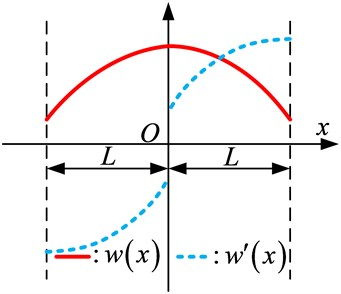Fig. 3An illustration of removal of possible discontinuities (convergence problem) at ends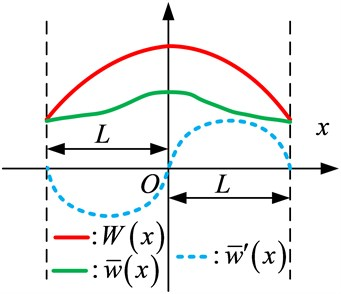Thus, the Fourier cosine series is able to correctly converge to $w\left(x\right)$ at any point over [0, $L$]. However, its first-derivative $w\mathrm{\text{'}}\left(x\right)$ is an odd function over $\left[-L,L\right]$ leading to a jump at end locations. The corresponding Fourier expansion of $w\mathrm{\text{'}}\left(x\right)$ continue on [0, $L$] and can be differentiated term-by-term only if $w\left(0\right)=w\left(L\right)=0$. Thus, its Fourier series expansion (sine series) will accordingly have a convergence problem due to the discontinuity at end points (Gibbs phenomena) when $w\mathrm{\text{'}}\left(x\right)$ is required to have up to the first-derivative continuity.

To overcome this problem, this Improved Fourier Series technique was proposed by Li [18, 19]. In this technique a new function $P\left(x\right)$ is considered in the displacement function:

9
$\stackrel{-}{w}\left(x\right)=W\left(x\right)+P\left(x\right)=\sum _{m=0}^{\mathrm{\infty }}{A}_{m}\mathrm{c}\mathrm{o}\mathrm{s}\left(\frac{m\pi x}{L}\right)+P\left(x\right),$

where the auxiliary function $P\left(x\right)$ in equation above represents an arbitrary continuous function that, regardless of boundary conditions, is always chosen to satisfy the following equations:

10
${P}^{\mathrm{\text{'}}}={w}^{\mathrm{\text{'}}}\left(0\right),\mathrm{}\mathrm{}\mathrm{}\mathrm{}\mathrm{}{P}^{\mathrm{\text{'}}}\left(L\right)={w}^{\mathrm{\text{'}}}\left(L\right),\mathrm{}\mathrm{}\mathrm{}\mathrm{}\mathrm{}\mathrm{}{P}^{\mathrm{\text{'}}\mathrm{\text{'}}\mathrm{\text{'}}}\left(0\right)={w}^{\mathrm{\text{'}}\mathrm{\text{'}}\mathrm{\text{'}}}\left(0\right),\mathrm{}\mathrm{}\mathrm{}\mathrm{}\mathrm{}\mathrm{}{P}^{\mathrm{\text{'}}\mathrm{\text{'}}\mathrm{\text{'}}}\left(L\right)={w}^{\mathrm{\text{'}}\mathrm{\text{'}}\mathrm{\text{'}}}\left(L\right).$

The actual values of the first and third derivatives (a sine series) at the boundaries need to be determined from the given boundary conditions. Essentially, $\stackrel{-}{w}\left(x\right)$ represents a residual beam function which is continuous over [0, $L$] and has zero slopes at the both ends, as shown in Fig. 3. Apparently, the cosine series representation of $\stackrel{-}{w}\left(x\right)$ is able to converge correctly to the function itself and its first derivative at every point on the beam.

Thus, based on the above analysis, $P\left(x\right)$ can be understood as a continuous function that satisfies Eq. (9), and its form is not a concern but must be a closed-form and sufficiently smooth over a domain [0, $L$] of the beam in order to meet the requirements provided by the continuity conditions and boundary constraints. Furthermore, it is noticeable that the auxiliary function $P\left(x\right)$ can improve the convergent properties of Fourier series.

### 2.2.2. Determining the expansion coefficients

Once the proper admissible function for the displacement field is selected Eq. (6), the next step is to find the expansion coefficients in the assumed displacement field. In order to do so Rayleigh-Ritz method is employed which is an energy-based method. To employ this method, it is necessary to state the potential and kinetic energies first in terms of displacement fields. The expression for the potential energy of the sector plate in local coordinates $\left(s,\varphi ,z\right)$ is derived from the constitutive laws and strain-displacement relations. According to the Mindlin plate theory. The strain energy of the annular sector plates can be expressed as:

11
${U}_{p}=\frac{1}{2}\underset{0}{\overset{\alpha }{\int }}\underset{0}{\overset{R}{\int }}D\left[\begin{array}{c}{\left(\frac{\partial {\theta }_{s}}{\partial s}\right)}^{2}+\frac{2\nu }{\left(s+a\right)}\frac{\partial {\theta }_{s}}{\partial s}\left(\frac{\partial {\theta }_{\varphi }}{\partial \varphi }+{\theta }_{s}\right)+\frac{1}{\left(s+a{\right)}^{2}}{\left(\frac{\partial {\theta }_{\varphi }}{\partial \varphi }+{\theta }_{s}\right)}^{2}\\ +\left(\frac{1-\upsilon }{2}\right)\frac{1}{\left(s+a{\right)}^{2}}{\left({\theta }_{\varphi }-\frac{\left(s+a\right)\partial {\theta }_{\varphi }}{\partial s}-\frac{\partial {\theta }_{s}}{\partial \varphi }\right)}^{2}\\ +{K}^{2}Gh\left[{\left(\frac{\partial {w}_{o}}{\partial s}+{\theta }_{s}\right)}^{2}+\frac{1}{\left(s+a{\right)}^{2}}{\left(\frac{\partial {w}_{o}}{\partial \varphi }+\left(s+a\right){\theta }_{\varphi }\right)}^{2}\right]\end{array}\right]\left(s+a\right)dsd\varphi ,$

the kinetic energy expression for annular sector plate is expressed as:

12
${T}_{p}=\frac{1}{2}{\omega }^{2}\underset{0}{\overset{\alpha }{\int }}\underset{a}{\overset{b}{\int }}\left(\rho h{{w}_{o}}^{2}+\frac{\rho {h}^{3}}{12}\left({{\theta }_{r}}^{2}+{{\theta }_{\varphi }}^{2}\right)\right)rdrd\varphi .$

The potential energy stored in the boundary springs is given by:

13
${U}_{sp}=\frac{1}{2}\left[\underset{0}{\overset{\alpha }{\int }}a{\left[{k}_{a}^{}{w}_{o}^{2}+{K}_{a}^{r}{{\theta }_{s}}^{2}+{K}_{a}^{t}{{\theta }_{\varphi }}^{2}\right]}_{{\mathrm{}}_{{\mathrm{}}_{s=0}}}+b{\left[{k}_{b}^{{\mathrm{}}^{}}{w}_{o}^{2}+{K}_{b}^{r}{{\theta }_{s}}^{2}+{K}_{b}^{t}{{\theta }_{\varphi }}^{2}\right]}_{s=R}\right]d\varphi$
$\mathrm{}\mathrm{}\mathrm{}\mathrm{}\mathrm{}\mathrm{}\mathrm{}+\frac{1}{2}\underset{0}{\overset{R}{\int }}\left[{\left[{k}_{0}^{}{w}_{o}^{2}+{K}_{0}^{r}{{\theta }_{s}}^{2}+{K}_{0}^{t}{{\theta }_{\varphi }}^{2}\right]}_{\varphi =0}+{\left[{k}_{\alpha }^{}{w}_{o}^{2}+{K}_{\alpha }^{r}{{\theta }_{s}}^{2}+{K}_{\alpha }^{t}{{\theta }_{\varphi }}^{2}\right]}_{\varphi =\alpha }\right]ds,$

where ${k}_{a}^{}$, ${k}_{b}^{}$ (${k}_{0}^{}$ and ${k}_{\alpha }^{}$) are linear spring constants, ${K}_{a}^{r}$, ${K}_{b}^{r}$ (${K}_{0}^{r}$ and ${K}_{\alpha }^{r}$) are rotational spring constants in radial direction, ${K}_{a}^{t}$, ${K}_{b}^{t}$ (${K}_{0}^{t}$ and ${K}_{\alpha }^{t}$) are rotational spring constants in tangential direction at edges $s=$0 and $s=R$ and $\varphi =$ 0 and $\varphi =\alpha$ respectively. All the classical homogeneous boundary conditions can be simply considered as special cases when the spring constants are either extremely large or substantially small. The units for the translational and rotational springs are N/m and Nm/rad, respectively.

After the potential and kinetic energies are expressed, then all the assumed displacement functions are inserted in the potential and kinetic energy equations and these equations are then further minimized with respect to the expansion coefficients in the displacement field. Mathematically, the Lagrangian for the annular sector plate can be generally expressed as:

14
$L={U}_{p}+{U}_{sp}-{T}_{p},$

where ${U}_{p}$ is strain energy of the plate, ${U}_{sp}$ is strain energy stored in the boundary springs and ${T}_{p}$ is the kinetic energy of the plate. Substituting Eq. (6) in Eqs. (11)-(13) and then minimizing Lagrangian Eq. (14) against all the unknown series expansion coefficients that is:

15
$\frac{\partial L}{\partial \mathrm{\Theta }}=0,\mathrm{}\mathrm{}\mathrm{}\text{where}\text{}\mathrm{\Theta }=\mathrm{}{A}_{mn},\mathrm{}{B}_{mn},\mathrm{}{C}_{mn},$

we can obtain a series of linear algebraic expressions in a matrix form as:

16
$\left(K-{\omega }^{2}M\right)E=0,$

where $E$ is a vector which contains all the unknown series expansion coefficients and $K$ and $M$ are the stiffness and mass matrices, respectively. $E$, $K$ and $M$ can be expressed as:

17
$E={\left\{\begin{array}{l}{A}_{-2,-2},{A}_{-2,-1},{A}_{-2,0},...,{A}_{m\text{'},-2},{A}_{m\text{'},-1},...{A}_{m\text{'},n\text{'}},...,{A}_{MN}\\ {B}_{-2,-2},{B}_{-2,-1},{B}_{-2,0},...,{B}_{m\text{'},-2},{B}_{m\text{'},-1},...{B}_{m\text{'},n\text{'}},...,{B}_{MN}\\ {C}_{-2,-2},{C}_{-2,-1},{C}_{-2,0},...,{C}_{m\text{'},-2},{C}_{m\text{'},-1},...{C}_{m\text{'},n\text{'}},...,{C}_{MN}\end{array}\right\}}^{T},$
18
$K=\left[\begin{array}{lll}{K}_{ii}^{p}& {K}_{ij}^{p}& {K}_{ik}^{p}\\ {K}_{ji}^{p}& {K}_{jj}^{p}& {K}_{jk}^{p}\\ {K}_{ki}^{p}& {K}_{kj}^{p}& {K}_{kk}^{p}\end{array}\right]+\left[\begin{array}{lll}{K}_{ii}^{sp}& {K}_{ij}^{sp}& {K}_{ik}^{sp}\\ {K}_{ji}^{sp}& {K}_{jj}^{sp}& {K}_{jk}^{sp}\\ {K}_{ki}^{sp}& {K}_{kj}^{sp}& {K}_{kk}^{sp}\end{array}\right],$
19
$M=\left[\begin{array}{ccc}{M}_{ii}& 0& 0\\ 0& {M}_{jj}& 0\\ 0& 0& {M}_{kk}\end{array}\right],$

where the subscripts $i$, $j$ and $k$ represents $w$, ${\theta }_{s}$ and ${\theta }_{\varphi }$ and the superscripts $p$ and $sp$ represents plate and boundary springs respectively. For conciseness, the detailed expressions for the stiffness and mass matrices are not shown here.

### 2.2.3. Determining the eigen values and eigen vectors

Once Eq. (16) is established, the eigenvalues (or natural frequencies) and eigenvectors of Mindlin annular sector plates can now be easily and directly determined from solving a standard matrix eigenvalue problem i.e. Eq. (16) using MATLAB. For a given natural frequency, the corresponding eigenvector actually contains the series expansion coefficients which can be used to construct the physical mode shape based on Eq. (6). Although this investigation is focused on the free vibration of Mindlin annular sector plate, the response of the annular sector plate to an applied load can be easily considered by simply including the work done by this load in the Lagrangian, eventually leading to a force term on the right side of Eq. (16).

## 3. Results and discussion

To check the accuracy and usefulness of the proposed technique, several numerical examples are presented in this section. It is important to mention here that the accuracy of the proposed method is greatly controlled by the number of truncation terms i.e. $M=N$, the more number of truncation terms we use we get more accurate results however the computational cost and time will increase with increasing number of truncation terms. Theoretically, there are infinite terms in the assumed displacement functions. However, the series is numerically truncated and finite terms are counted in actual calculations which will be further explained in the text to follow. Moreover, in identifying the boundary conditions in this section, letters C, S, and F have been used to indicate the clamped, simply supported and free boundary condition along an edge, respectively. Therefore, the boundary conditions for a plate are fully specified by using four alphabets with the first one indicating the B.C. along the first edge, $r=a$. The remaining (the second to the fourth) edges are ordered in the counterclockwise direction.

First of all, in order to check the accuracy and usefulness we first consider a fully clamped Mindlin annular sector plate. Fully clamped (CCCC) boundary conditions can easily be achieved by setting the stiffnesses of the restraining springs to an infinitely large number (1014) in the numerical calculations. The first six non-dimensional frequency parameter, $\mathrm{\Omega }=\omega {b}^{2}{\left(\rho h/D\right)}^{1/2}$ are tabulated in Table 1 along with the reference results from  and .

Table 1First six non dimensional frequency parameter Ω=ωb2ρh/D1/2 for fully clamped (CCCC) Mindlin Annular sector plates (ϕ=2π/3, a/b= 0.25, h/b= 0.2)

 $M=N$ Mode sequence 1 2 3 4 5 6 2 31.481 42.907 62.894 66.199 73.800 94.776 4 31.084 41.872 56.108 62.435 72.906 75.775 6 31.059 41.823 55.972 62.411 71.269 72.862 8 31.054 41.813 55.951 62.406 71.148 72.852 10 31.053 41.810 55.946 62.405 71.125 72.849 12 31.053 41.809 55.943 62.404 71.118 72.847 14 31.053 41.809 55.942 62.404 71.116 72.847 Ref.  31.056 41.814 55.951 62.420 71.127 72.862 Ref.  31.057 41.814 55.951 62.420 71.127 72.862

Similarly, in Table 2, first six non-dimensional frequency parameter for Mindlin annular sector plate having simply supported radial edges and clamped circumferential edges (CSCS) boundary conditions has been given along with the reference results from [9, 27]. A good agreement in the present values and reference values can be observed.

Table 2First six non dimensional frequency parameter Ω=ωb2ρh/D1/2 for Mindlin Annular sector plates (ϕ=π/3, a/b= 0.5, h/b= 0.1) having simply supported radial edges and clamped circumferential edges (CSCS)

 $M=N$ Mode sequence 1 2 3 4 5 6 2 77.625 104.054 161.254 170.932 194.112 243.145 4 76.567 102.955 149.795 166.952 190.467 215.603 6 76.476 102.765 149.410 166.772 190.093 206.703 8 76.449 102.696 149.323 166.726 189.961 206.315 10 76.439 102.667 149.290 166.710 189.908 206.228 12 76.435 102.654 149.275 166.703 189.883 206.196 14 76.432 102.647 149.267 166.699 189.871 206.182 Ref.  76.902 103.682 150.413 167.327 191.593 207.276 Ref.  76.902 103.682 150.413 167.327 191.593 207.276

Next we consider annular sector plate simply supported at radial edges and having different combination of boundary conditions (free-clamped, free-simply supported, simply supported-simply supported, simply supported-free and clamped-free) at the circumferential edges. The simply supported condition is simply produced by setting the stiffnesses of the translational and rotational springs to $\infty$ and 0, respectively, and the free edge condition by setting both stiffnesses to zero. The fundamental frequency parameters with different boundary conditions are shown in Table 3. The current results agree well with those taken from references [28, 29].

Next to illustrate the convergence and numerical stability of the current solution procedure, several sets of results for fully clamped Mindlin annular sector plates having different sector angles and using different truncation numbers ($M=N=$2, 4, 6, 8, 10, 12, 14) are presented in Tables 4-7. Furthermore, the fast convergence pattern can also be observed in Fig. 4.

Table 3Fundamental frequency parameter Ω=ωb2ρh/D1/2 for Mindlin Annular sector plates having simply supported radial edges and different boundary conditions at circumferential edges (a/b= 0.5)

 Sector angle ($\varphi$) Thickness to radius ratio ($h/b$) Method Boundary conditions at circumferential edges F-C F-S S-S S-F C-F 195 0.1 Present 19.998 10.224 38.365 4.560 12.696 Ref.  19.999 10.227 38.636 4.675 12.680 Ref.  20.097 10.239 38.764 – – 0.2 Present 17.503 9.130 32.508 4.005 11.413 Ref.  17.582 9.366 32.871 4.542 11.427 Ref.  17.764 9.396 33.190 – – 210 0.1 Present 19.620 9.685 38.222 4.507 12.678 Ref.  19.610 9.664 38.455 4.584 12.659 Ref.  19.706 9.675 38.582 – – 0.2 Present 17.235 8.681 32.419 3.997 11.417 Ref.  17.294 8.877 32.734 4.458 11.425 Ref.  17.294 8.904 33.050 – – 270 0.1 Present 18.654 8.213 37.868 4.392 12.639 Ref.  18.622 8.130 38.010 4.372 12.615 Ref.  18.715 8.139 38.134 – – 0..2 Present 16.548 7.450 32.200 3.999 11.433 Ref.  16.566 7.546 32.394 4.263 11.430 Ref.  16.739 7.567 32.704 – –

A fast convergence pattern can be observed in the tabulated results as well as Fig. 4, therefore it can be concluded that sufficiently accurate results can be obtained with a small number of terms in the series expansion and the solution is consistently refined as more and more terms are included in the series expansion.

Table 4First five non-dimensional frequency parameters Ω=ωb2ρh/D1/2 for CCCC Mindlin annular sector plates (a/b= 0.6, h/b= 0.1)

 $M=N$ Sector angle Mode sequence 1 2 3 4 5 2 $\varphi =\frac{\pi }{6}$ 145.584 240.451 251.723 331.721 391.843 4 144.104 237.420 249.040 328.660 350.364 6 144.032 237.301 248.941 328.487 349.816 8 144.020 237.280 248.924 328.452 349.739 10 144.017 237.274 248.920 328.442 349.720 12 144.016 237.272 248.918 328.438 349.713 14 144.015 237.271 248.917 328.436 349.711

Table 5First five non-dimensional frequency parameters Ω=ωb2ρh/D1/2 for CCCC Mindlin annular sector plates (a/b= 0.6, h/b= 0.1)

 $M=N$ Sector angle Mode number 1 2 3 4 5 2 $\varphi =\frac{\pi }{2}$ 104.250 116.563 163.387 223.325 232.424 4 102.977 112.649 130.467 173.504 220.564 6 102.911 112.460 129.978 155.396 187.319 8 102.900 112.420 129.896 154.985 185.972 10 102.897 112.408 129.871 154.895 185.781 12 102.896 112.403 129.862 154.865 185.726 14 102.895 112.401 129.857 154.852 185.705

Table 6First five non-dimensional frequency parameters Ω=ωb2ρh/D1/2 for CCCC Mindlin annular sector plates (a/b= 0.6, h/b= 0.1)

 $M=N$ Sector angle Mode number 1 2 3 4 5 2 $\varphi =\frac{2\pi }{3}$ 102.797 109.453 139.708 221.999 227.027 4 101.566 106.400 115.457 143.396 194.321 6 101.503 106.239 115.077 128.766 147.504 8 101.492 106.203 115.006 128.447 146.310 10 101.489 106.192 114.984 128.368 146.140 12 101.488 106.188 114.975 128.339 146.089 14 101.488 106.186 114.970 128.327 146.068

Table 7First five non-dimensional frequency parameters Ω=ωb2ρh/D1/2 for CCCC Mindlin annular sector plates (a/b= 0.6, h/b= 0.1)

 $M=N$ Sector angle Mode sequence 1 2 3 4 5 2 $\varphi =\frac{7\pi }{6}$ 101.717 103.774 115.254 220.887 222.496 4 100.537 101.885 104.287 114.756 138.302 6 100.480 101.779 104.088 107.716 113.103 8 100.470 101.753 104.042 107.560 112.462 10 100.467 101.744 104.026 107.512 112.360 12 100.467 101.741 104.019 107.492 112.325 14 100.466 101.739 104.015 107.482 112.308

From Tables 4-7 it can be seen that when the truncated numbers change from $M×N=$ 10×10 to 12×12, the maximum difference of the frequency parameters does not exceed 0.003 for the worst case, which is acceptable. Furthermore, in modal analysis the natural frequencies for higher order modes tend to converge slower as compared to the lower order modes which can easily be observed in Fig. 4 that the 9th mode frequency converges slowly as compared to the 6th and 3rd mode. Thus a suitable truncation number should be used to achieve the accuracy of the largest desired natural frequency. In view of above and excellent numerical behavior of the current solution, the truncation number for all subsequent calculations in the present method is taken as $M=N=$12.

Next we study the effect of sector angle and thickness-radius ratio and cutout ratio on non-dimensional frequency parameter. The effect has been graphically represented in Figs. 5-7, respectively.

Fig. 4Convergence pattern of frequency parameters with no. of terms (M=N)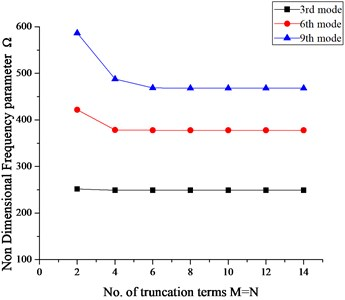Fig. 5Effect of sector angle on non-dimensional frequency parameter ‘Ω’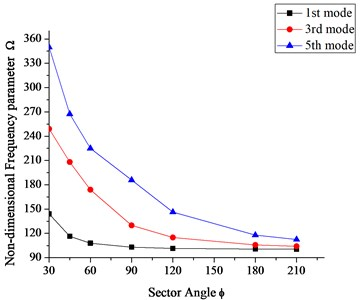Fig. 6Effect of thickness to radius ratio (h/b) on the frequency parameter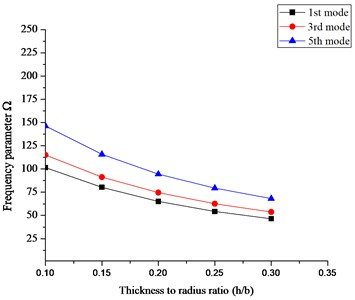Fig. 7Effect of cutout ratio (a/b) on the frequency parameters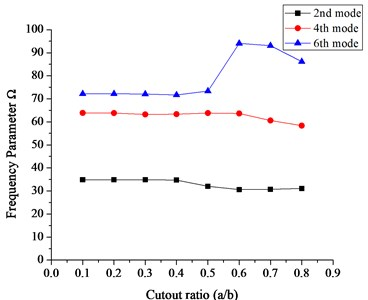It can be seen in Fig. 5 that for smaller sector angles i.e. $\varphi \le 2\pi /3$, the decrease in the frequency parameters is more as compared to the sector angles greater than $2\pi /3$. Similarly, in figure 6, 1st, 3rd and 5th mode frequency parameters have been plotted against different thickness to radius ratios ($h/b$) for a fully clamped (CCCC) Mindlin annular sector plate having sector angle $=2\pi /3$ and cutout ratio $=a/b=$ 0.6. It can be observed that with the increase in thickness to radius ratio the frequency parameter always decreases. Similarly, the effect of cutout ratio i.e. inner radius to outer radius ($a/b$) on the frequency parameters for a FSFS mindlin annular sector plate having sector angle $=\pi /3$, and $h/b=$ 0.2 can be seen in Fig. 7.

As mentioned previously, using this method, a Mindlin circular sector plate can also be analyzed easily just by equating the inner radius of Mindlin annular sector plate to zero without modifying the equations or the solution algorithm. Table 8 shows first six non-dimensional frequency parameter along with reference results for Mindlin circular sector plates having inner radius $=a=$0.0001, different thickness to radius ratio and sector angles and subjected to simply supported radial edges and clamped circular edge (SCS) boundary condition respectively. A close agreement can be observed in the present values and the reference results.

Table 8First six non-dimensional frequency parameters for SCS Mindlin Circular sector plates (a/b= 0.0001)

 Sector angle ($\varphi$) Thickness to radius ratio $\left(h/b$) Method Mode sequence 1 2 3 4 5 6 $\frac{\pi }{6}$ 0.1 Present 91.419 148.357 205.117 208.046 278.356 281.097 Ref.  93.450 152.630 206.900 213.080 274.650 283.190 0.2 Present 66.272 98.992 131.454 131.981 161.648 163.749 Ref.  67.933 102.560 132.860 135.610 165.680 167.820 $\frac{\pi }{2}$ 0.1 Present 31.657 60.112 71.009 92.956 110.151 118.760 Ref.  32.205 60.637 72.221 93.450 111.320 120.450 0.2 Present 26.298 46.323 53.227 67.436 77.370 82.512 Ref.  26.993 46.906 54.466 67.933 78.582 83.903 $\pi$ 0.1 Present 20.778 31.969 45.586 54.582 60.614 71.698 Ref.  20.223 32.205 45.773 53.859 60.637 72.221 0..2 Present 17.752 26.630 36.470 42.169 46.726 53.813 Ref.  17.773 26.993 36.758 42.393 46.906 54.466 $\frac{7\pi }{6}$ 0.1 Present 19.915 28.398 39.635 51.986 52.898 65.557 Ref.  19.489 28.599 39.786 51.998 52.416 65.091 0.2 Present 16.871 23.958 32.269 40.788 40.916 49.836 Ref.  17.092 24.293 32.519 41.067 41.195 49.859

All the examples mentioned above are limited to different combinations of classical boundary conditions which are viewed as special case of elastically restrained edges. After verifying the convergence, accuracy and effectiveness of the proposed method for different combinations of classical boundary conditions, the method is further employed here to study the vibration characteristics of Mindlin annular sector and circular sector plates subjected to general elastic boundary conditions.

In order to simulate the elastic boundary conditions, it is important to study the effect of restraining springs first on the frequency parameters so that proper value to the restraining springs could be assigned. Fig. 8 shows the effect of restraining springs stiffness on the frequency parameter for Mindlin annular sector plate ($a/b=$ 0.6, $h/b=$ 0.2 and $\varphi =$120).

Fig. 8 shows 1st, 5th and 10th mode frequency parameters plotted against the spring stiffnesses by varying the stiffnesses of one group of boundary spring from 0 to 1016 while keeping the stiffnesses of the other group equal to infinite i.e 1016. It can be seen in Fig. 8(a) that the frequency parameter almost remains at a level when the stiffness of the translational spring in $z$ direction is less than 108 and greater than 1012 where as other than this range the frequency parameter increases with increasing stiffness values. Similar phenomena can be observed in case of rotational spring stiffness however a slight change in frequency parameter can be observed with in the stiffness range from 108 to 1010. Based on the analysis it can be concluded that stable frequency parameter can be obtained when the stiffnesses for all the restraining springs is more than 1012 or less than 108 and also it is suitable and valid to use the stiffness value 1014 to simulate the infinite stiffness in the numerical calculations since the frequency parameter remain at the same level for values greater than equal to 1014.It can also be concluded that the elastic stiffness range for translational spring is more than the two rotational springs.

Fig. 8a) Effect of translational spring stiffness (k) on Ω, and effect of rotational spring stiffness b) in tangential direction (Kt) on Ω, c) in radial direction (Kr) on Ω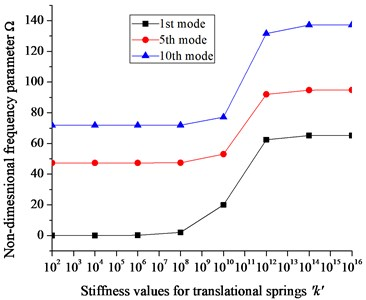a)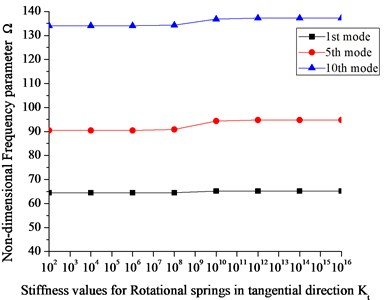b)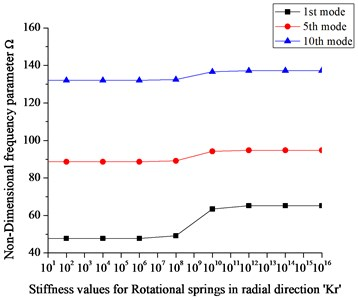c)

From Fig. 8, an elastic boundary condition can easily be defined with any stiffness value between 108 to 1012. To the author’s best knowledge, no reported results are available in literature for vibration analysis of Mindlin annular sector plates under general elastic boundary conditions. As mentioned earlier the present method can be used to obtain natural frequency parameters for Mindlin annular sector plates under general elastic boundary condition regardless of modifying solution algorithm and procedure.

In order to achieve valuable results for annular sector plates subjected to elastic boundary conditions, we define an elastic restraint ‘E1having corresponding translational and rotational spring stiffness values $k=$1e8, ${K}_{r}=$0 and ${K}_{t}=$0. Tables 9-11 shows first five natural frequency parameters for Mindlin annular sector plates having different sector angles and thickness-radius ratio ($h/b$) subjected to E1E1E1E1, FE1 FE1 and CE1CE1 boundary conditions where E1E1E1E1, FE1 FE1 and CE1CE1 represents the combination of classic and elastic boundary conditions at edges $a$ and $b$ as well as $\varphi =$ 0 and $\alpha$.

Due to unavailability of results in previous literature for these types of boundary conditions (E1E1E1E1, FE1 FE1 and CE1CE1), the comparison has been made with results obtained from ABAQUS. However, we know that the core algorithm of the ABAQUS software is based on the finite element method. Furthermore, the FEM computational accuracy strongly depends on the size of the mesh and the type of element selection. For more accuracy in the higher frequency region and for complex geometries, a highly refined mesh and a higher order finite element is needed. We know that the smaller the mesh size, the greater the number of elements we get for analysis which further requires more computer memory and subsequently a high computational cost. Since the geometry under investigation in this manuscript is a simpler geometry therefore a simple free meshing technique with mesh size 0.005 and S4R (Shell 4 node Reduced Integration) element type has been used which is computationally inexpensive and is considered suitable for this type of geometry and modal analysis. Keeping the mesh size 0.005, the number of elements used in the analysis for annular sector plate having sector angle $\pi$/3, $\pi$/2, 2$\pi$/3 and $\pi$ are 13040, 20080, 29727 and 40240 respectively.

A very good agreement can be observed in Tables 9-11 between the calculated results and the one obtained from ABAQUS. This shows that the present method can be easily applied to classical and elastic type boundary conditions as well as their combination without modifying the solution algorithm and procedure. The results tabulated in Tables 9-11 can be used as a bench mark for future computational methods.

Table 9First five natural frequency parameters for Mindlin Annular sector plates subjected to E1E1E1E1 type elastic boundary conditions (a/b= 0.6)

 Sector angle ($\varphi$) Thickness to radius ratio ($h/b$) Method Natural frequency modes 1 2 3 4 5 $\frac{\pi }{3}$ 0.1 Present 337.934 435.140 506.867 1520.326 1966.903 ABAQUS 337.690 437.350 507.130 1522.500 1969.600 0.2 Present 241.050 302.391 330.646 2587.184 3074.622 ABAQUS 240.970 303.960 330.420 2590.100 3080.200 $\frac{\pi }{2}$ 0.1 Present 314.276 389.282 479.851 870.662 1294.086 ABAQUS 313.770 390.700 480.060 872.380 1295.400 0.2 Present 225.655 274.476 318.276 1411.322 1991.271 ABAQUS 225.400 275.570 318.090 1412.500 1994.500 $\frac{2\pi }{3}$ 0.1 Present 300.548 361.101 440.420 703.848 884.878 ABAQUS 301.310 364.010 443.800 707.710 901.740 0..2 Present 216.209 257.039 302.020 1032.654 1342.753 ABAQUS 216.710 259.290 303.580 1037.500 1374.000 $\pi$ 0.1 Present 288.597 325.207 378.969 516.005 622.710 ABAQUS 288.110 325.230 380.110 517.160 622.670 0.2 Present 206.659 235.054 270.651 650.104 811.858 ABAQUS 206.290 235.350 271.530 650.900 811.980

Table 10First five natural frequency parameters for Mindlin Annular sector plates subjected to FE1FE1 type elastic boundary conditions (a/b= 0.4)

 Sector angle ($\varphi$) Thickness to radius ratio ($h/b$) Method Natural frequency 1 2 3 4 5 $\frac{\pi }{3}$ 0.1 Present 119.832 264.818 319.661 1483.220 1916.028 ABAQUS 119.940 264.970 319.570 1484.100 1917.400 0.2 Present 83.397 180.756 222.669 2577.428 3060.712 ABAQUS 83.395 180.750 222.710 2580.000 3066.200 $\frac{\pi }{2}$ 0.1 Present 57.023 251.087 267.380 786.925 1222.649 ABAQUS 56.935 251.210 267.440 787.520 1223.700 0.2 Present 41.696 179.512 185.090 1389.419 1971.191 ABAQUS 40.802 179.550 184.680 1390.300 1974.300 $\frac{2\pi }{3}$ 0.1 Present 24.833 201.973 271.669 561.948 806.253 ABAQUS 24.039 202.070 271.430 562.430 806.550 0.2 Present 18.952 149.812 189.712 995.529 1318.058 ABAQUS 17.523 149.840 189.290 995.930 1319.700 $\pi$ 0.1 Present 3.867 120.269 233.439 411.437 434.108 ABAQUS 3.856 120.260 233.370 411.390 434.060 0.2 Present 3.869 105.112 171.054 610.370 758.047 ABAQUS 0.000 105.100 170.900 610.820 758.160

## 4. Conclusion

An improved Fourier series method has been presented for vibration analysis of moderately thick annular and circular sector plates with classical and general elastic restraints along its edges. Regardless of the boundary conditions, the displacement function is invariantly expressed as an improved trigonometric series which converges uniformly at an accelerated rate. The efficiency, accuracy and reliability of the present method have been fully demonstrated by various numerical examples for moderately thick annular sector plates having different cutout ratios and sector angles.

Table 11First five natural frequency parameters for Mindlin Annular sector plates subjected to CE1CE1 type elastic boundary conditions (a/b= 0.4)

 Sector angle ($\varphi$) Thickness to radius ratio ($h/b$) Method Natural frequency modes 1 2 3 4 5 $\frac{\pi }{3}$ 0.1 Present 5161.242 5329.372 6024.050 7500.647 9771.648 ABAQUS 5182.600 5349.600 6044.000 7521.400 8046.600 0.2 Present 6628.586 6823.656 7744.270 9710.654 12411.918 ABAQUS 6665.600 6859.200 7778.100 9746.900 10565.000 $\frac{\pi }{2}$ 0.1 Present 5167.620 5242.262 5540.684 6140.785 7122.635 ABAQUS 5188.700 5261.800 5560.000 6158.100 7141.200 0.2 Present 6633.347 6724.016 7105.490 7936.324 9269.663 ABAQUS 6673.500 6762.800 7142.300 7970.700 9306.600 $\frac{2\pi }{3}$ 0.1 Present 5171.158 5212.374 5378.060 5694.631 6205.673 ABAQUS 5191.900 5231.300 5397.000 5711.500 6222.000 0..2 Present 6636.080 6687.398 6895.770 7327.756 8047.005 ABAQUS 6676.100 6726.100 6933.100 7362.200 8080.800 $\pi$ 0.1 Present 5175.030 5192.529 5265.762 5397.220 5599.831 ABAQUS 5195.000 5210.600 5284.500 5414.600 5616.400 0.2 Present 6639.126 6661.568 6752.481 6926.255 7207.997 ABAQUS 6678.800 6699.900 6790.300 6961.700 7241.800

The effect of sector angle, thickness to radius ratio and restraining springs on the frequency parameters has been discussed. The present method is also employed to study the vibration analysis of moderately thick circular sector plates without modifying the solution procedure. Results for moderately thick annular sector plates under general elastic boundary conditions for various thicknesses to radius ratio and sector angle are presented which can serve as a bench mark for future computational methods. The accuracy of the results has been verified by comparing it with those available in literature and with ABAQUS. An excellent agreement is observed between the results obtained using the present method and with those available in literature. Keeping in view the accuracy and fast convergence behavior this method can easily be further extended to study the vibration analysis of various built up structures without modifying the solution algorithm and procedure.

23 December 2015
Accepted
20 May 2016
Published
30 September 2016
SUBJECTS
Modal analysis
Keywords
vibrations
circular plates
annular plates
sector plates
natural frequency
mode shapes
arbitrary boundary conditions
Acknowledgements

The authors gratefully acknowledge the financial support from the National Natural Science Foundation of China (No. U1430236) and Natural Science Foundation of Heilongjiang Province of China (No. E2016024).